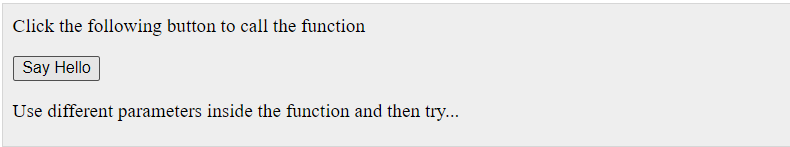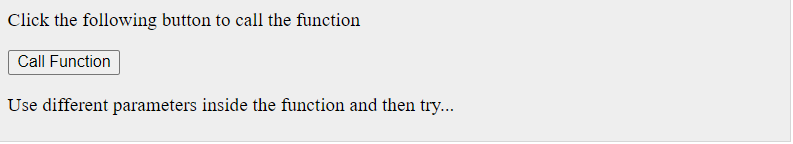top of page
Search

# How to declare functions in Javascript?A function is a group of reusable code which can be called anywhere in your program. This eliminates the need of writing the same code again and again. It helps programmers in writing modular codes. Functions allow a programmer to divide a big program into a number of small and manageable functions.

There are mainly two advantages of JavaScript functions.

1. Code reusability: We can call a function several times so it save coding.

2. Less coding: It makes our program compact. We don’t need to write many lines of code each time to perform a common task.

### Syntax

The basic syntax is shown here.

```<script type = "text/javascript">
<!--
function functionname(parameter-list) {
statements
}
//-->
</script>

```

### Example

Try the following example. It defines a function called sayHello that takes no parameters −

```<script type = "text/javascript">
<!--function sayHello()
{
}
//-->
</script>```

## Calling a Function

To invoke a function somewhere later in the script, you would simply need to write the name of that function as shown in the following code.

```<html>
<script type = "text/javascript">
function sayHello() {
document.write ("Hello there!");
}
</script>

<body>
<p>Click the following button to call the function</p>
<form>
<input type = "button" onclick = "sayHello()" value = "Say
Hello">
</form>
<p>Use different text in write method and then try...</p>
</body>
</html>```

### Output## Function Parameters

Till now, we have seen functions without parameters. But there is a facility to pass different parameters while calling a function. These passed parameters can be captured inside the function and any manipulation can be done over those parameters. A function can take multiple parameters separated by comma.

### Example

Try the following example. We have modified our sayHello function here. Now it takes two parameters.

```<html>
<script type = "text/javascript">
function sayHello(name, age) {
document.write (name + " is " + age + " years old.");
}
</script>

<body>
<p>Click the following button to call the function</p>
<form>
<input type = "button" onclick = "sayHello('Zara', 7)"
value = "Say Hello">
</form>
<p>Use different parameters inside the function and then try...
</p>
</body>
</html>```

### Output## The return Statement

A JavaScript function can have an optional return statement. This is required if you want to return a value from a function. This statement should be the last statement in a function.

For example, you can pass two numbers in a function and then you can expect the function to return their multiplication in your calling program.

### Example

Try the following example. It defines a function that takes two parameters and concatenates them before returning the resultant in the calling program.

```<html>
<script type = "text/javascript">
function concatenate(first, last) {
var full;
full = first + last;
return full;
}
function secondFunction() {
var result;
result = concatenate('Zara', 'Ali');
document.write (result );
}
</script>

<body>
<p>Click the following button to call the function</p>
<form>
<input type = "button" onclick = "secondFunction()" value
= "Call Function">
</form>
<p>Use different parameters inside the function and then try...
</p>
</body>
</html>```

### OutputSource: Tutorlapoint

The Tech Platform